Next: Terms of the Cost Up: Nonlinear Independent Component Analysis Previous: Model Structure

# Cost Function

The goal is to estimate the posterior pdf of all the unknown variables of the model. This is done by ensemble learning which amounts to fitting a simple, parametric approximation to the actual posterior pdf . The cost function C is the misfit between the approximation and the actual posterior and is measured by the Kullback-Leibler information which is sensitive to the probability mass of densities. This is the most important advantage over maximum a posteriori (MAP) estimation which is computationally less expensive but is sensitive to probability density, not mass. This is why MAP estimation suffers from overfitting, which would be a serious problem since there are so many estimated variables, while ensemble learning is able avoid it.

For the time being, let us denote the set of all observations vectors x(t) by X and denote all the other parameters by a vector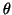. The actual posterior pdf is thus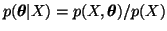. The joint pdf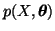is obtained from the definition of the model in (3)-(14) and p(X) is a normalising factor which does not depend on the unknown variables.

Let us denote the approximation of the posterior pdf by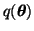. In order for the cost function to be computable in practice, a simple factorial form needs to be chosen for the approximation. The maximally factorial form would be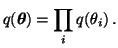(15)

Notice that we have used the usual notation with probability density functions where q with different arguments are taken to be different functions.

The assumption of factorialis equivalent to assuming the unknown variables independent given the observations. This is not true, of course, but we have to make this approximation in order to obtain a practical algorithm. The only exception to this maximally factorial form is that the index Mi(t) of the Gaussian and the corresponding source si(t) are allowed to have posterior dependency, that is, the terms q(Mi(t), si(t)) are not further factorised.

The approximation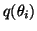should be chosen so that it fits the actual posterior as closely as possible. This is accomplished by choosingto be Gaussian for other variables than sources and for sources choosing q(Mi(t), si(t)) = Q(Mi(t)) q(si(t) | Mi(t)), where q(si(t) | Mi(t)) is Gaussian.

Let us denote the mean and variance ofby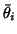and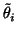, respectively. The result of learning is then an estimate of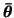and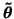which tell the posterior mean and variance of all the unknown variables.

The term p(X) is constant with respect to the unknown parameters. Instead of the pure Kullback-Leibler information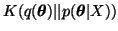it is therefore possible to use the following cost function: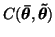=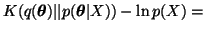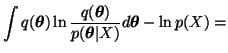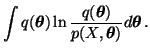(16)

Notice that the variables Mi(t) are discrete and those terms are summed over, not integrated over, in the Kullback-Leibler information. For simplicity this is omitted from (16).

Due to simple factorial forms ofandthe cost function splits into simple terms which are easy to compute. Consequently, it is also easy to differentiate the cost function with respect toandand use the derivatives for constructing the learning algorithm.Next: Terms of the Cost Up: Nonlinear Independent Component Analysis Previous: Model Structure
Harri Lappalainen
2000-03-03